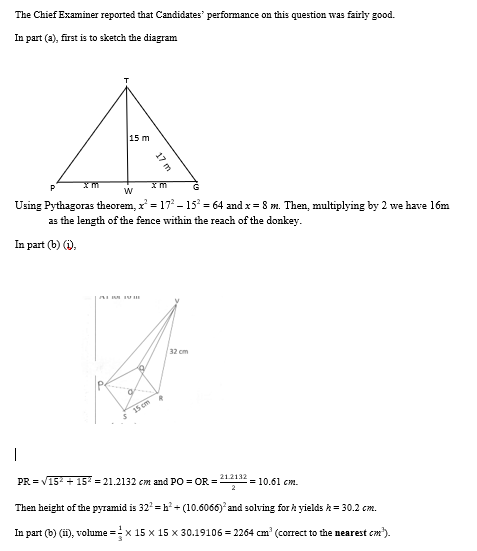### General Mathematics Paper 2, WASSCE (PC), 2018

Question 9

1. A donkey is tied with a rope to a post which is 15 m from a fence. If the length of the rope between the donkey and the post is 17 m, calculate the length of the fence within the reach of the donkey.
2. The base of a right pyramid with vertex, V, is a square, PQRS, of side 15 cm. If the slant height is 32 cm long:
3. represent the information in a diagram;
4. calculate its:
5. height, correct to one decimal place;
6. volume, correct to the nearest cm3.

### Observation